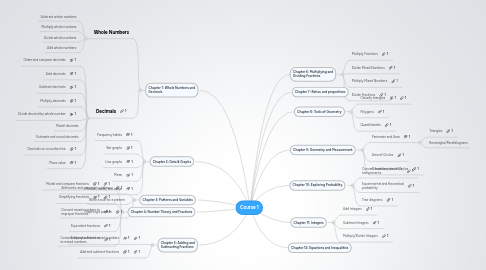# Course 1

Get Started. It's FreeCourse 1## 1. Chapter 1: Whole Numbers and Decimals

### 1.1. Whole Numbers

1.1.1. Subtract whole numbers

1.1.2. Multiply whole numbers

1.1.3. Divide whole numbers

### 1.2. Decimals

1.2.1. Order and compare decimals

1.2.3. Subtract decimals

1.2.4. Multiply decimals

1.2.5. Divide decimal by whole number

1.2.6. Model decimals

1.2.7. Estimate and round decimals

1.2.8. Decimals on a number line

1.2.9. Place value

## 9. Chapter 9: Geometry and Measurement

### 9.1. Perimeter and Area

9.1.1. Triangles

9.1.2. Rectangles/Parallelograms